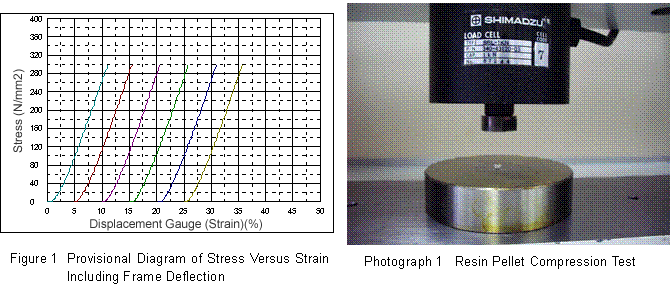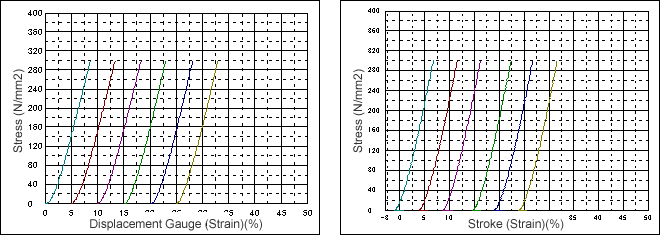# Measurement of the Compressive Elastic Modulus of Resin Pellets with a Precision Universal Tester Using Trapezium-X

The data obtained for evaluating the strength of materials using a precision universal tester includes tension, compression, bending strength, and the elastic modulus. This report describes an attempt to measure the compressive elastic modulus of resin pellets of a variety of shapes and sizes. Here, the elastic modulus is measured in three ways; (1) provisional elastic modulus measurement including frame deflection without using an external displacement gauge; (2) reliable elastic modulus measurement with a displacement gauge between compression plates; and (3) elastic module measurement using the deflection correction function provided by TRAPEZIUM-X, the materials testing operational software. The elastic modulus is measured between 100 N/mm2 and 150 N/mm2, the linear portion of the stress-strain curve's elasticity region (in the vicinity of the inflexion point). The effectiveness of the deflection correction provided by TRAPEZIUM-X, the materials test operational software, was confirmed by comparing the 3 elastic moduli.
Fig. 1 is a provisional diagram of stress versus strain including the frame deflection, without using an external displacement gauge. Fig. 2 is a diagram of stress versus strain using a displacement gauge between compression plates. Fig. 3 is a diagram of stress versus strain using the deflection correction function of TRAPEZIUM-X, the materials test operational software.Here the compressive strength measurements were performed at a speed of 1 mm/min, using the Shimadzu Autograph AG-1kNZ and a compression test jig.
As shown in Table 1, the elastic modulus obtained using the deflection correction provided by TRAPEZIUM-X, the materials test software, is comparable to that when deflection has been measured reliably. Further, as shown in Table 2, the elastic modulus can be measured by TRAPEZIUM-X even for samples with comparatively small modulus values.

Table 1 Elastic Modulus Comparison for Resin Pellet 1

 Measurement Method Average Value of Elastic Modulus MPa Provisional Elastic Modulus Including Frame Deflection 3177.02

Figure 2:Diagram of Stress Versus Strain Using Displacement Gauge Between Compression Plates
Figure 3:Diagram of Stress Versus Strain Using Deflection Correction

 Elastic Modulus From Displacement Gauge Between Compression Plates 4259.03 Elastic Modulus From Materials Software Deflection Correction 4280.66

Table 2 Elastic Modulus Comparison for Resin Pellet 2

 Measurement Method Average Value of Elastic Modulus MPa Provisional Elastic Modulus Including Frame Deflection 295.1 Elastic Modulus From Displacement Gauge Between Compression Plates 302.2 Elastic Modulus From Materials Software Deflection Correction 302.9

From this, values close to the true elastic modulus can be obtained through correction calculations using the Shimadzu Autograph AG-1kNZ and TRAPEZIUM-X materials test software, even when measurement of the actual sample deformation is difficult.